# Search Results (9)

View
Selected filters:
• Scott Burns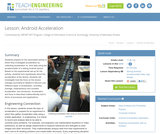Conditions of Use:
Rating

Students prepare for the associated activity in which they investigate acceleration by collecting acceleration vs. time data using the accelerometer of a sliding Android device. Based on the experimental set-up for the activity, students form hypotheses about the acceleration of the device. Students will investigate how the force on the device changes according to Newton's Second Law. Different types of acceleration, including average, instantaneous and constant acceleration, are introduced. Acceleration and force is described mathematically and in terms of processes and applications.

Subject:
Engineering
Physics
Material Type:
Lesson Plan
Provider:
TeachEngineering
Provider Set:
TeachEngineering
Author:
Brian Sandall
Scott Burns
09/18/2014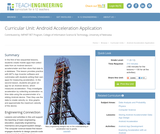Conditions of Use:
Rating

In the first of two sequential lessons, students create mobile apps that collect data from an Android device's accelerometer and then store that data to a database. This lesson provides practice with MIT's App Inventor software and culminates with students writing their own apps for measuring acceleration. In the second lesson, students are given an app for an Android device, which measures acceleration. They investigate acceleration by collecting acceleration vs. time data using the accelerometer of a sliding Android device. Then they use the data to create velocity vs. time graphs and approximate the maximum velocity of the device.

Subject:
Computer Science
Engineering
Material Type:
Full Course
Unit of Study
Provider:
TeachEngineering
Provider Set:
TeachEngineering
Author:
Brian Sandall
Scott Burns
09/18/2014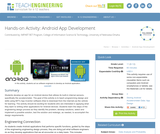Conditions of Use:
Rating

Students develop an app for an Android device that utilizes its built-in internal sensors, specifically the accelerometer. The goal of this activity is to teach programming design and skills using MIT's App Inventor software (free to download from the Internet) as the vehicle for learning. The activity should be exciting for students who are interested in applying what they learn to writing other applications for Android devices. Students learn the steps of the engineering design process as they identify the problem, develop solutions, select and implement a possible solution, test the solution and redesign, as needed, to accomplish the design requirements.

Subject:
Engineering
Material Type:
Activity/Lab
Provider:
TeachEngineering
Provider Set:
TeachEngineering
Author:
Brian Sandall
Scott Burns
09/18/2014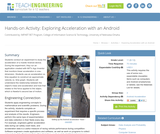Conditions of Use:
Rating

Students conduct an experiment to study the acceleration of a mobile Android device. During the experiment, they run an application created with MIT's App Inventor that monitors linear acceleration in one-dimension. Students use an acceleration vs. time equation to construct an approximate velocity vs. time graph. Students will understand the relationship between the object's mass and acceleration and how that relates to the force applied to the object, which is Newton's second law of motion.

Subject:
Computer Science
Engineering
Material Type:
Activity/Lab
Provider:
TeachEngineering
Provider Set:
TeachEngineering
Author:
Brian Sandall
Scott Burns
09/18/2014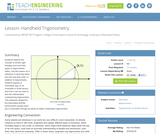Conditions of Use:
Rating

Students explore the concept of similar right triangles and how they apply to trigonometric ratios. Use this lesson as a refresher of what trig ratios are and how they work. In addition to trigonometry, students explore a clinometer app on an Android® or iOS® device and how it can be used to test the mathematics underpinning trigonometry. This prepares student for the associated activity, during which groups each put a clinometer through its paces to better understand trigonometry.

Subject:
Engineering
Mathematics
Trigonometry
Material Type:
Lesson Plan
Provider:
TeachEngineering
Provider Set:
TeachEngineering
Author:
Scott Burns
09/18/2014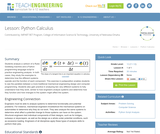Conditions of Use:
Rating

Students analyze a cartoon of a Rube Goldberg machine and a Python programming language script to practice engineering analysis. In both cases, they study the examples to determine how the different systems operate and the function of each component. This exercise in juxtaposition enables students to see the parallels between a more traditional mechanical engineering design and computer programming. Students also gain practice in analyzing two very different systems to fully understand how they work, similar to how engineers analyze systems and determine how they function and how changes to the system might affect the system.

Subject:
Engineering
Education
Mathematics
Trigonometry
Material Type:
Lesson Plan
Provider:
TeachEngineering
Provider Set:
TeachEngineering
Author:
Brian Sandall
Scott Burns
09/18/2014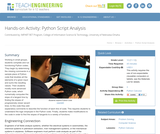Conditions of Use:
Rating

Working in small groups, students complete and run functioning Python codes. They begin by determining the missing commands in a sample piece of Python code that doubles all the elements of a given input and sums the resulting values. Then students modify more advanced Python code, which numerically computes the slope of a tangent line by finding the slopes of progressively closer secant lines; to this code they add explanatory comments to describe the function of each line of code. This requires students to understand the logic employed in the Python code. Finally, students make modifications to the code in order to find the slopes of tangents to a variety of functions.

Subject:
Engineering
Education
Mathematics
Trigonometry
Material Type:
Activity/Lab
Provider:
TeachEngineering
Provider Set:
TeachEngineering
Author:
Brian Sandall
Scott Burns
09/18/2014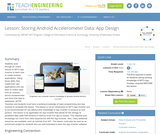Conditions of Use:
Rating

Students work through an online tutorial on MIT's App Inventor to learn how to create Android applications. Using those skills, they create their own applications and use them to collect data from an Android device accelerometer and store that data to databases. NOTE: Teachers and students must have a working knowledge of basic programming and App Inventor to complete this lesson. This lesson is not an introduction to MIT's App Inventor and is not recommended for use without prior knowledge of App Inventor to produce an end product. This lesson is an application for App Inventor that allows for the storage of persistent data (data that remains in memory even if an app is closed). This required prior knowledge can come from other experiences with the App Inventor. Also, many additional resources are available, such as tutorials from MIT. This lesson could also be used as an enrichment project for students who are self-motivated to learn the App Inventor software.

Subject:
Engineering
Material Type:
Lesson Plan
Provider:
TeachEngineering
Provider Set:
TeachEngineering
Author:
Brian Sandall
Scott Burns
09/18/2014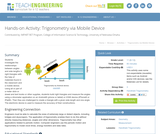Conditions of Use:
Rating

Students investigate the relationships between angles and side lengths in right triangles with the help of materials found in the classroom and a mobile device. Using all or part of a meter stick or dowel and text books or other supplies, students build right triangles and measure the angles using a clinometer application on an Android® (phone or tablet) or iOS® device (iPhone® or iPad®). Then they are challenged to create a triangle with a given side length and one angle. The electronic device is used to measure the accuracy of their constructions.

Subject:
Engineering
Mathematics
Trigonometry
Material Type:
Activity/Lab
Provider:
TeachEngineering
Provider Set:
TeachEngineering
Author:
Scott Burns